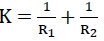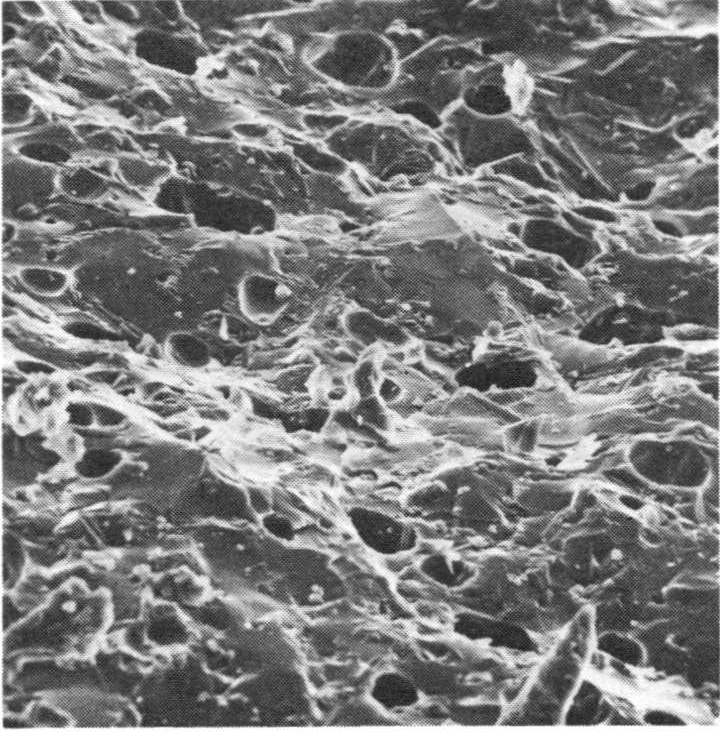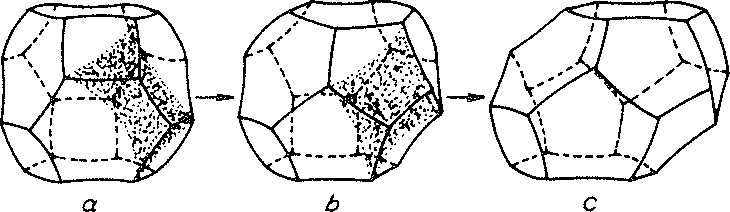Mikrostruktur
und mechanisches Verhalten des Schaumglases

von Dr. sc. techn. ETH Rudolf Trinkner

A.1. The strucutre of foam glass

A.1.1. Terms (1)

The word “foam” is a collective term used for two fundamentally different types of foam, namely for “spherical foam” and for “polyhedron foam”..

“Spherical foam” is a concentrated distribution or accumulation of independent spherical bubbles in a gaseous, liquid or solidified distribution medium. Each bubble in the spherical foam possesses its own capillary curvature pressure, which is proportional to the inverse of the curvature radius. Through expansion or growth within a limited space the spherical bubbles are forced to deform in a viscous or elastoplastic medium into polyhedron honeycomb bubbles, without losing their independence (“false polyhedron foam”).).

In contrast to spherical foam with individual bubbles, “polyhedron foam” is a composite of polyhedral shaped bubbles, which have lost their independence. In this bubble composite, a certain equilibrium structure is created caused by capillary forces acting in different spatial directions, and which can only be sustained by a very specific polyhedron form of the bubbles.

In this paper we will deal with solidified foams, which have been expanded from liquid glass melts.

For this reason using the terms above, we can define the following foam glass types:

• 1)Spherical foam glass and
• 2)polyhedron foam glass.

A.1.2. Physical fundamentals for understanding the morphology of polyhedron foam glass

The structure of the foam is governed by the equilibrium principle of mechanics. It is equivalent to the principle of minimum surface energy.

J. Plateau formulated the following

basic principles for polyhedron foams of liquid solutions in 1873 in his book "Statique experimentale et theorique des liquides":

• a)Only three films can meet in a line, and they must intersect at identical angles of 120° (equilibrium of surface forces or principle of minimum surface energy)1).1).
• b)Only six films can meet at a point and neighbouring boundary lines (“edges”) must intersect at identical angles of 109° 28' (consequence of a).).
• b)Each cell wall between two cells must have a constant average curvature• to b) If more than six films were to meet, the equilibrium would become unstable (information from 3. Plateau an Kelvin).

• The film between two neighbouring bubbles of a liquid foam in a state of equilibrium is loaded at a given point by the surface loading Δρ=2⋅α⋅K.

DWith thisis the mean curvature at the point in question (R1 and R2 the associated curvature radii, which have different signs if the central points of the curvature lie on different sides of the cell wall film) and α the surface tension of the liquid film.

• 1)Films are understood here as the cell walls of the polyhedron foams.

Conclusions:

• 1)D1) The wall films between two given bubbles of a polyhedron foam have a constant surface loading Δρ=Δρo auf. Thus this film also has the constant mean curvature(= principle c) of J. Plateau.).
• 2)2) The wall films of a weightless polyhedron foam unconstrained on all sides which is in a state of equilibrium and made of a given liquid solution all have the mean curvaturesince Δρ disappears everywhere; i.e. all bubbles have the same internal pressure ρ.

A.1.3. Microscopic observations on foam glass

A.1.3.1. Spherical foam glass

Fig. No. 3 shows the electron microscope image of the fracture surface of a spherical foam glass sample made of sintered coloured waste glass powder (0≤Φ≤125μ), whose density is ρ=1.75gr / cm3 . During sintering the maximum temperature is held for 11 minutes at 960°C.Fig No. 3. Fracture surface of a spherical foam glass sample at scale 392 : 1

Using X-ray investigation it was possible to determine that the sample under consideration here contained approximately 10 + 15 % devitrite and traces of cristobalite1) . Based on a thin microsection, the mean free distance of the spherical bubbles of the spherical foam glass sample shown here was determined at 80 μ .

A.1.3.2. Polyhedron foam glass

An analysis of the section of the polyhedron foam glasses produced by us generally shows the structure represented in Fig. No. 4.Fig No. 4  section of a coarse-celled polyhedron foam glass sample

1) SiO2. is polymorphous and for the reason occurs naturally in three crystalline forms:

• 1)as  quartz
• 2)as cristobalite (cubic) and
• 3)as tridymite (hexagonal).

This is a section of the same foam glass cuboid as shown in Fig. No. 1. Here you can see the richness of forms of the innumerable polyhedron cell bubbles!

In order to express that the cell walls are comprised of fixed shape and low capillary space spherical foam glass, in future we will refer to them as films 1).

Images of microsections through a given polyhedron foam glass sample using polarised light show that the glass base material of the cell wall films contain many small embedded crystals as well as chemically unreacted expanding agent.

Since in the original glass melt - from which the polyhedron foam glass is expanded - surface tensions are active, the physical principles for liquid polyhedron foams, as described in section 1.2, can also be used for the solidified polyhedron foam glass.  For this reason it can be asserted that in statistical mean the morphology of all given two-dimensional sections through a random polyhedron foam glass sample can be expressed using the following idealised sketch - in accordance with the principles of J. Plateau.Idealised section through a polyhedron foam glass sample

• 1) E. Manegold understands a film to be a fixed-form low-capillary space sheet, whereas he understands a membrane to be a fixed-form high-capillary space sheet   (1).

We also see that at points where three cell wall films normally meet with the equilibrium angle of 120°, the surfaces are rounded cylindrically with so-called plateau edges.

A.1.4. Archetypes for the elementary polyhedron of the cellular structure of real polyhedronfoam glasses

The centre surfaces of all cell wall films of the polyhedron foam glass form a series of surfaces which is called a cell structure. It is formed from the surfaces of the most diverse convex polyhedrons which completely pervade the space according to the rules. These bodies will be referred to hereafter as elementary polyhedron or base polyhedron of the cell structure of the polyhedron foam glass!1)

The morphology is based on the idea that the diverse world of shapes of the elementary polyhedra of the cell structure of a given polyhedron foam glass sample are only derivatives of one archetype (2). According to their school of thought it would now be necessary to search for the archetypes of all individual elementary polyhedra of the cell structure of the polyhedron foam glass.

The following requirements are placed on the archetypes of the base polyhedron of the cell structure of the polyhedron foam glass:

• 1)It must be possible to form ideal foam glasses from many congruent archetypal cell structures of ideal foam glasses, whose morphologies accord with the basic principles for liquid foams of J. Plateau;
• 2)The congruent archetypes must completely pervade the space (consequence of 1);;
• 3)The statistical mean of the percentage distribution of the four-, five- and six-sided partial surfaces of the cell structure of such an ideal foam glass should ideally equate to observations from nature.
• 1)The expression “base polyhedron or elementary polyhedron of the polyhedron foam glass” is intended to mean the same thing.

A.1.4.1. The α- tetradecahedron of W. Kelvin as an archetype of the elementary polyhedron of real polyhedron foam glass

Fig. No. 5a shows the α - tetradecahedron of W. Kelvin (3), which can be adopted as the base polyhedron of an ideal polyhedron foam glass.Fig. No. 5  The α - tetradecahedron and the β - tetradecahedron

This polyhedron is closely related to the truncated octahedron1). It has eight double curved hexagonal surfaces and six square flat surfaces. All 36 edge lines of the α- tetradecahedron are congruent flat curves. The curved surfaces (minimum surfaces) are a requirement of the minimum of the surface energy.

In a polyhedron foam glass, whose cell structure is composed of many such identical elementary polyhedra, three cell wall films meet at an intersection angle of 120° at an “edge”, one is four-sided and two are hexagonal. This ideal foam glass meets all the conditions of J. Plateau.

The polyhedron forms the basis for the model polyhedron foam glasses “TOP” and “ORTHO” which are dealt with later.

A.l.4.2. The β - tetradecahedron of R.E. Williams as an archetype of the elementary polyhedron of the real polyhedron foam glass

Matzke and Nestler showed that the static distribution of the polyhedron surfaces of the cell structure of soap bubble packing differs significantly  from that of ideal foams, which have cell structures, which are comprised of many

• 1)The truncated octahedron belongs to the thirteen Archimedean bodies.

Kelvin polyhedra. Thus they identified a preference for pentagonal side surfaces.

The following summary from R.E. Williams is intended to illustrate this:

Counts per polyhedron surface

600 identically shaped bubbles 0.1 or 0.2cm3

%
1OO

small bubbles

0.05 cm3 in mixture

%
50

large bubbles 0.4cm3 in mixture

%
Ideal foams of
α-

%
β-

%
3
4 10.5 32.9 11.3
 42.9 14.3
5 67.0 58.1 48.1
 57.1
6 22.1 8.9 28.3
 57.1 28.6
7 4.4 0.1 11.2
8     1.0
9     0.1

For this reason R.E. Williams in 1968 suggested the β - tetradecahedron as the base polyhedron for the cell structure of natural foams (4)!

Fig. No. 5c shows the new polyhedron of R.E. Williams, which can be viewed as the elementary polyhedron of an ideal polyhedron foam glass consistent with the base principles of J. Plateau. It can be mechanically derived from the Kelvin polyhedron, by taking one “edge” of two of its neighbouring six-sided surfaces - including the “edges” connecting at its ends - (see Fig. 5a), turning the whole thing and joining it together again. The resulting polyhedron (Fig. No. 5b) with four four-sided, four five-sided and six six-sided surfaces can also pervade the space. The same operation is carried out with the corresponding group of “edges” on the opposite side of the polyhedron. This leads to the body (β -tetradecahedron) on Fig. No. 5c.

This transformation retains the same number of 14 surfaces, 24 vertices and 36 “edges” as the α - tetradecahedron and the corner angles remain 109°28'. As the table above show, the percentage distribution of the surfaces corresponds roughly to the conditions in nature.

This base polyhedron is used later to design the elementary polyhedron of the model foam glass.

Nach oben springen
Stereological and volumetric investigations